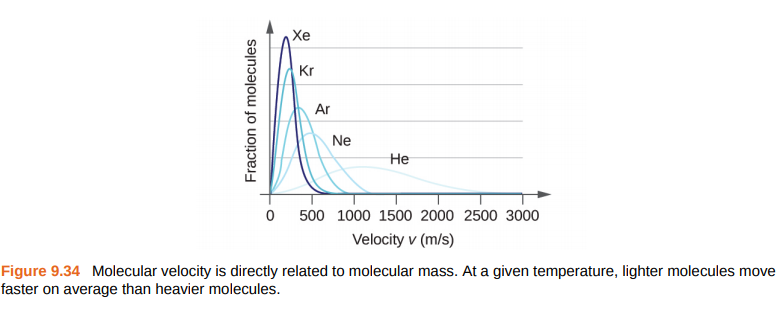# Problem: The distribution of molecular velocities in a sample of helium is shown in Figure 9.34. If the sample is cooled, will the distribution of velocities look more like that of H2 or of H2O? Explain your answer.

###### FREE Expert Solution

We’re being asked to determine if the sample of Helium is cooled, will the distribution of velocities look more like that of H2 or of H2O.

Recall that gases behave most ideally at low pressures and high temperatures.

In the graph, the y-axis is the relative number of particles while the x-axis is the molecular speed. We can think of the molar mass of a gas as being related to the number of particles ( molar mass =  particles).

The molecular speed of a gas is given by:

82% (60 ratings)###### Problem Details

The distribution of molecular velocities in a sample of helium is shown in Figure 9.34. If the sample is cooled, will the distribution of velocities look more like that of H2 or of H2O? Explain your answer.Frequently Asked Questions

What scientific concept do you need to know in order to solve this problem?

Our tutors have indicated that to solve this problem you will need to apply the Velocity Distributions concept. You can view video lessons to learn Velocity Distributions. Or if you need more Velocity Distributions practice, you can also practice Velocity Distributions practice problems.

What professor is this problem relevant for?

Based on our data, we think this problem is relevant for Professor Hove's class at RUTGERS.

What textbook is this problem found in?

Our data indicates that this problem or a close variation was asked in Chemistry - OpenStax 2015th Edition. You can also practice Chemistry - OpenStax 2015th Edition practice problems.